# Yield of Soya Paste (Okara) Influenced by Some Mechanical Parameters of an Automated Grain Drinks Production Machine

Gana IM1* and Gbabo A2

1Agricultural & Bio-Environmental Engineering Department, Federal Polytechnic Bida, Nigeria

2Agricultural & Bioresources Engineering Department, Federal University of Technology, Nigeria

Received Date: June 24, 2019;  Published Date: July 10, 2019

#### Abstract

The effect of mechanical parameters of an automated grain drinks processing machine on yield of paste (okara) was investigated in this study. Response Surface Methodology (RSM) was used to develop polynomial regression model and investigate the effect of blending blade, basket orientation and speed of rotation on yield of paste in the process of extracting soymilk from soybean. A three factors-five levels central composite rotatable design (CCRD) was used in the study. The result of the experiment revealed that blade type and speed of rotation have significant (p≤0.05) effect on yield of the paste while basket orientation has insignificant (p≤0.05) effect. It was observed that the experimental data fitted better with coefficient of determination R value of 0.9828, coefficient of correlation R-Squared value of 0.9658. The Predicted R-Squared of 0.940884 was in reasonable agreement with the Adjusted R-Squared of 0.935095. The value of adequate Precision of 19.38 also showed that the model equation can be used to navigate within the experimental range. Numerical optimization carried out with the goal of maximizing the yield of paste revealed optimum values of 5-blade assembly, basket orientation of 30° and speed of 1000rpm for consistency of 7.88 with a desirability of 0.928. The blade type and the speed of rotation were the most influential variables affecting the yield of paste. The result of this study provided standard input machine variables capable of yielding paste (okara) at various operating condition to meet the consumer’s desire.

#### Introduction

Soya bean paste (okara) is the by-product obtained after extraction of aqueous milk from soya bean. Large quantities of the paste are generated as by-product obtain the process of extracting the aqueous liquid from soya beans and in the production process of tofu. Several research works have reported on quantity of okara to be generated from soya beans. According to Liu , 1.1 pounds of okara can be generated from 1 pound of soya beans, while Riaz , stated that 1 kilogram of soybeans used for soymilk processing can generates between 1.4-1.8kg of okara. According to Africa Do Business Limited (2012), 1.2kg of fresh okara is produced from 1 kg of soya beans processed for manufacturing of tofu .

Okara was reported to have wide range of benefits, which includes excellent and abundant nutrient for livestock production, pet food and food product. Its high level of extenders and abundant nutrient makes it useful in variety of food products. It is used as food ingredient more especially in soup, salad and vegetables dishes and it has almost the same form and texture as coconut. The major constituents of okara are carbohydrate, protein, lipid and fiber. The nutrient content of okara depends mainly on soymilk processing procedure and the efficiency with which soymilk is extracted from the wet pulp. Also, the quantity of okara produced depends on the efficiency of the milling and extraction method Aguado . This paper is a presentation of investigation of the interrelationship of the functional parameters of an automated grains drink production machine with yield of paste(okara). The machine was developed in the Agricultural and Bioresources Engineering department of the Federal Univesity of Technology, Minna.

##### Soya bean samples preparation

Twenty-five kilograms of soya beans of TGX 1954-IFXTGX 1835-10E variety was sourced from Minna central Market Niger State Nigeria. It was sorted, cleaned and washed with tap water to remove foreign materials before dividing into twenty samples of 1 kg each. Each of the samples was soaked separately in water for 12hrs at room temperature of 28°C as recommended by John and May . The soaked soybean’s husk was removed by means of the pressure of two hands and cleaned with continuous flow of fresh water. Then the clean dehulled samples were processed using the grain processing machine based on the design matrix shown in (Table 1).

Table 1: Matrix Transformation of Five Level- Three Factors Central Composite Rotatable Design of the Experiment.##### Equipment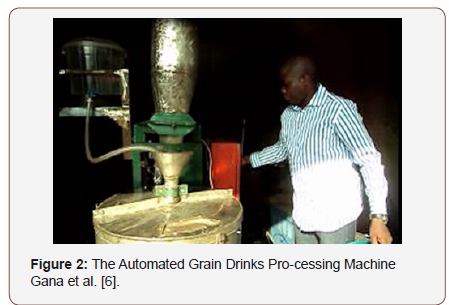The gearbox controls the movement of the upper and lower arms. It is responsible for the opening and the closing of the blending chamber, engagement, and disengagement of the basket from rotation. Programmable timer was used as the control system and was designed in such a way that the operating time can easily be varied. The control system consists of the following units; power and pre-set units, erasable programmable read-only memory, a display unit, and the relay unit. The electric motors used by the machine are 2hp and 5hp electric motors. The 2hp powered the gearbox while the 5hp powered the central shaft and the centrifugal basket Gana et al.  (Figure 2-4).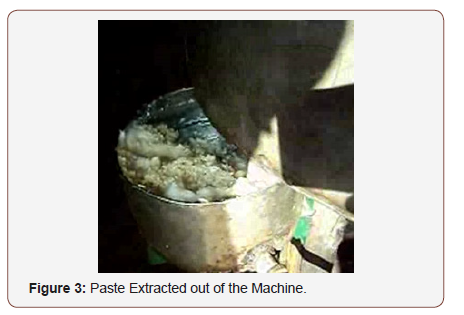##### Experimental design

A five-level, three factor CCRD was applied and the total number of experiments were 20 (2k+2k+6); where k is the number of independent variables Anounye . Blade type, basket orientation and speed of blending were the independent variables selected to optimize the yield of okara (residue). The experimental design matrix is presented in (Table 2).

Table 2: Regresional Analysis of Response of Yield of the Paste.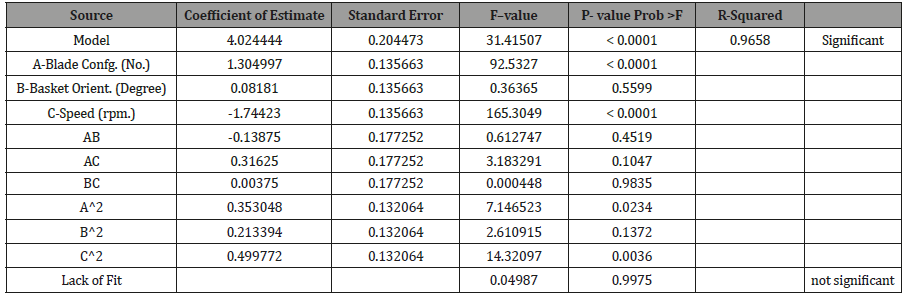##### Statistical analysis

The Design Expert (7.0.0) software package was used for the regression and graphical analysis of the data. The okara yield was taken as the response of the design experiment. The experimental data obtained by the above procedure was analyzed by the response surface regression Aworanti et al.  using the following second-order polynomial equation (1) where; y is the response (Okara yield (%), xi and xj are the uncoded independent variables, i and j are the linear and quadratic coefficients respectively, β° is the regression co-efficient, g is the number of factors studied and optimized in the experiment. Statistical analysis of the model equation and evaluation of the analysis of variance (ANOVA) was carried out. Confirmatory experiments were also performed to validate the equation.##### Conducting the experiment

The experiment was conducted as per design matrix shown in (Table 1). The automated grain drinks processing machine was switched on and setting were inputted (blending and sieving time) after which the soaked soya beans was feed into the machine. As blending operation commences the water valve was open and water began to flow into the system. Soya milk were washed and filtered into bottom of the drum from where they flow out through the liquid outlet. This operation continuous until the blending time elapsed, immediately the time elapses the paste was expelled out of the machine. The quantity of Okara produced was determined as described by Onuorah et al.  is reported in equation 1.

#### Yield of the paste

This is the quantity of paste produced by the machine and was determined as reported by Onuorah et al. It is given as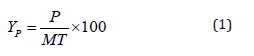where, YP =the Yield of the Paste (%)

P = the amount of the material not able to pass through the sieve (kg)

MT = the total weight of the material fed into the machine (kg)

##### Yield of the paste

The yield of the paste is percentage ratio of weight of the paste to total weight of material fed into the machine and it was evaluated using the formula reported by Onuorah et al. and is given equation 1. The effects of independent variables; blade configuration, basket orientation and speed on the yield of the paste was presented in (Table 1). The highest yield of the paste of 8.37% was obtained when the soya beans sample was processed with 4 blade assembly, basket with half angle of 40o and speed of 864rpm while the least yield of the paste of 1.65% was obtained when the sample was processed with 3 blade assembly, basket with half angle of 30° and speed of 1400rpm

##### Statistical analysis

The result of the statistical analysis of variance (ANOVA) of the experimental is presented in (Table 2). The effects, contribution, model coefficient, test for Lack-of-fit and the significance of the variables and their respective interaction on the yield of the paste were also presented in the table. Quadratic model was statistically significant for the response. The significant model terms were identified at 95% significance level. The Quadratic regression model equation developed to predict yield of the paste with respect to functional machine parameters (independent variables) were given as shown in equations 1.

##### Calibration of the model

The model F-value of 31.42 implies that the model was significant. There was only 0.01% chance that a Model F value this large could occurred due to noise. The value of Probability >F less than 0.0500 indicated that model terms were significant. In this case A, C and A2 were significant model terms with P-values of <0.0001, <0.0001, 0.0234 and 0.0036 respectively. It can be clearly observed that A had more significant effect on yield of the paste with coefficient of estimate of 1.305 followed by C (Table 2).

The “Lack of Fit F-value” of 0.05 implies that the Lack of Fit was not significant relative to the pure error. There was 99.75% chance that a “Lack of Fit F-value” this large could occur due to noise. Nonsignificant lack of fit is good. The coefficient of determination R value of 0.9828 indicated that the model was able to predict 98.28 % of the variance and only 1.72% of the total variance was not explained by the model. The coefficient of correlation R-Squared value of 0.9658 was high very close. The Predicted R-Squared of 0.940884 was in reasonable agreement with the Adjusted R-Squared of 0.935095 which indicated that the experimental data fitted better Xin and Saka . Adequate Precision of 19.38 is above the desirable minimum value of 4 was reported by Salam et al. . This indicated that the model can be used to navigate the design space.

The regressed yield of paste model is shown in equation 1

YP = 4.02+1.30A+0.082B-1.74C-0.14AB+0.32AC+3.750×10- 3BC+0.35A2+0.21B2+0.50C2 (1)

where, YP =Yield of Paste (%)

C = Speed of Blending (rpm.)

The model equation was improved by removing insignificant model terms. Values greater than 0.1000 implies that the model terms are not significant (that is A, AB, AC, BC, ABC, B2sub>, A2B, A2C, AB2, AC2, B2C, BC2C,>, A3, B3 and C3 are not significant) and since these terms are insignificant the model was reduced to equation 2 Aworanti et al. .

The fitted yield of paste model is given in equation 4.6

YP = 4.02+1.30A-1.74C+0.35A2 (2)

It is important to add that the variable A in the model has positive co-efficient implying a direct proportionality while C has negative co-efficient implying an indirect proportionality. That is independent increase in A increased the yield of the paste while increase in C decreased the yield of the paste.

##### Simulation and validation of the model

The model equation obtained was simulated and the yield of the paste was observed to be within the experimental range. From (Table 1) the yield of the paste was observed to be in close agreement with the predicted value. This is ascertation between the predicted value and the observed value validating the need for the model equation to be used to determine the optimum yield of the paste at various operating conditions within the experimental range.

The response surface and contour plot for yield of paste. The yield of the paste decreased from 4.3% to 0.75% with increased in speed of blending from 1000rpm to 1400rpm (Figure 5,6). This could be due to increase in impact, cutting and shearing actions of the blade with increased in speed of blending which resulted to decrease in yield of the paste. This is in line with the result of an earlier report by Jayesh  where rotational speed was found to be a key factor to size reduction of solid materials. Where higher speed of blending resulted to more segregation of materials (less yield of paste) than lower speed of blending resulted to lesser segregation of materials (more yield of paste). On the order hand the yield of the paste increased from 4.2% to 7.4% with increased in blade number from 3 blades to 5 blades assembly. This could be as result of decreased in contact between the blade and the grains with increased in configuration which caused less segregation of the materials.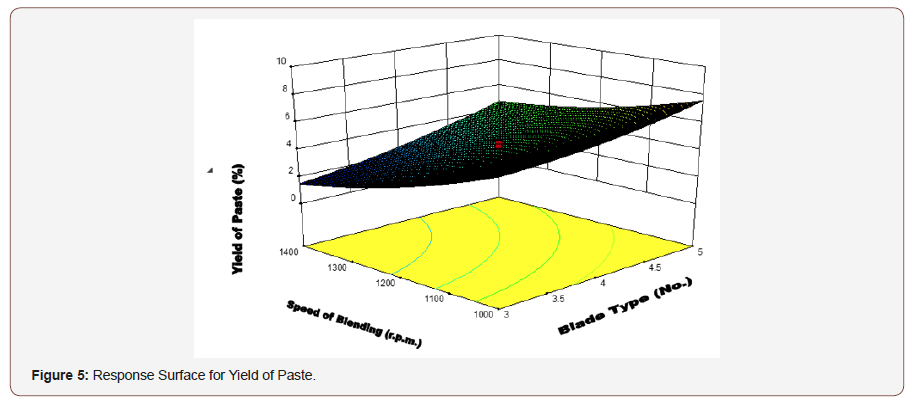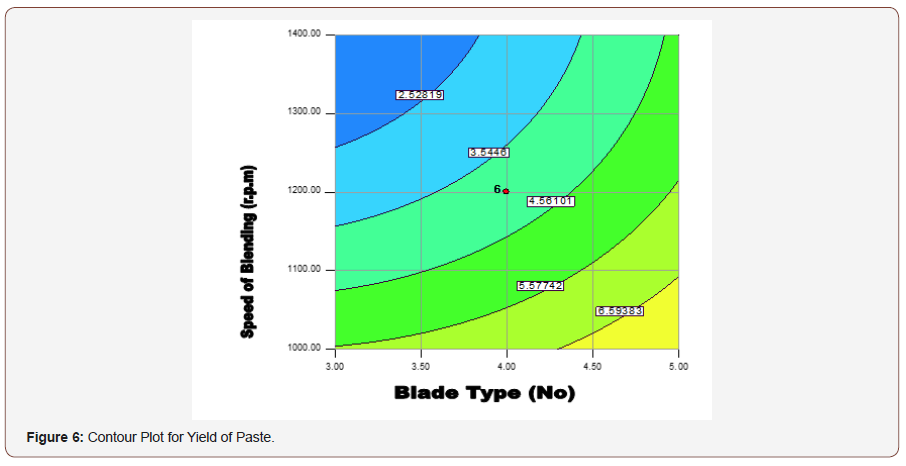This result agreed with the result of earlier findings by Gbabo et al.  where blade design was found to affect segregation of materials. It can be clearly observed that there was significant (p≤0.05) difference of 3.55% in yield of the paste between speed of 1000 rpm and 1400 rpm. Also, significant (p≤0.05) difference of 3.2% was observed in yield of the paste as the blade configuration increased from 3 and 5 blades assembly. The minimum yield of the paste of 0.74% was obtained from combination of speed of 1400rpm and 3 blades assembly. This value was observed to increase to 2.37% with increased in speed of blending to 1536rpm. This could as result losing of some of the finer particles of the milk together with the paste. Douglas  reported that higher speed of blending produced finer particles that clogged together and blocked the sieve holes, thus prevent materials from passing through the holes.

##### Relationship between basket orientation and yield of okara

The basket orientation was observed to have no significant (P≤0.05) effect on the yield of okara (paste). Also, from (Figure 7) there was not any significant (P≤0.05) difference between 3-blade assembly and 5-blade assembly. This is clear indication that the basket has no effect on yield of okara (Figure 7).##### Optimization of the machine functional parameters

The ramp for the optimization of machine functional parameters; blade type, basket orientation and speed with goal of maximizing the yield of the paste (okara) as criteria is shown in Figure 8; it gave the optimum values of 5-blades assembly, basket of half angle of 30°and speed of 1000rpm, while for the response was yield of okara of 7.88% and desirability of 0.928 (Figure 8).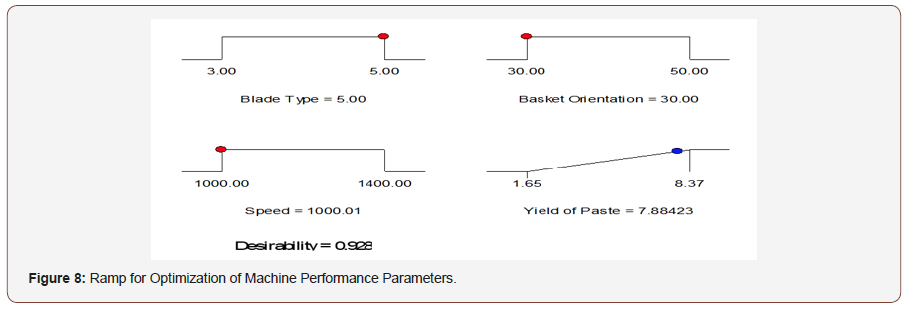#### Conclusion

The interaction effects between the machine parameters showed that yield of paste (okara) decrease with increased in speed of rotation from 1000rpm to 1400rpm and increase with increased in blade number from 3 to 5 blade assembly. The basket orientation was found to have no significant effects on yield of the yield of paste (okara).

The developed mathematical models and individual co-efficient were found to be significant while the Lack of fit was significant. The experimental values were found to fit better with close agreement between predicted r-squared and adjusted r-squared values. The model equations can be used to navigate within the experimental ranges with high adequate precision values of 19.38.

Optimization of the functional machine parameters was carried out using numerical optimization technique by applying desirability function method in rsm. The best optimal machine functional parameters of 5-blades assembly, basket of half angle of 30 o and speed of 1000rpm., while for the yield of paste (okara) was 7.88% and desirability of 0.928.

None.

#### Conflict of Interest

No conflict of interest.

Article Details
Citation
Keywords
Scroll to
Scroll to Top